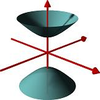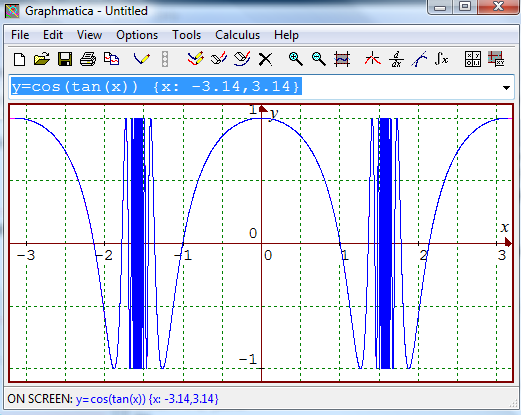#### You may also like### A Close Match

Can you massage the parameters of these curves to make them match as closely as possible?### Prime Counter

A short challenge concerning prime numbers.### The Right Volume

Can you rotate a curve to make a volume of 1?

# Trigger

##### Age 16 to 18 ShortChallenge Level

We are looking to sketch $y=\cos(\tan(x))$.

What do we know about this? Certainly we will have $-1\leq y\leq 1$ since $\cos(x)$ is always between $-1$ and $1$, regardless of its arguament.

Next, we know that $\cos(\theta)$ is zero for the values $\theta = (2n+1)\frac{\pi}{2}$ and has turning points inbetween these values at $m\pi$

Next, we know that $\tan{x}$ has asymptotes at $x = \pm \frac{\pi}{2}, \pm\frac{3\pi}{2}$. Only two of these lie in the range $-\pi \leq x \leq \pi$. This allows us to see that $\tan x$ takes every real number value exactly twice on the range $-\pi < x \leq \pi$ and is undefined at two points on this range.

Thus, $y=\cos(\tan x)$ will have an infinite number of turning points on the specified range, and these occur precisely at the values
$$0, \pm\tan^{-1}(\pi), \pm \tan^{-1} (2\pi), \pm \tan^{-1}(3\pi) \dots$$
Numerically, these values are
$$0, \pm 1.262627, \pm 1.412965, \pm 1.465089, \pm 1.491386, \pm 1.50720, \pm 1.517794, \dots$$
Note that these values tend to the numbers $\pm \frac{\pi}{2}$, which are the two values for which our function is undefined

Similarly, zeros will occur at the values
$$\pm\tan^{-1}\left(\frac{\pi}{2}\right),\tan^{-1}\left(\frac{3\pi}{2}\right), \tan^{-1}\left(\frac{5\pi}{2}\right)\dots$$

Putting all this together we obtain a bounded function which oscillates infinitely often in any interval containing the point $\frac{\pi}{2}$ or the point -$\frac{\pi}{2}$

The final part is to determine which points are maxima and which are minima. This is easily done by evaluating $\cos(\tan(0)) = +1$ and $\cos(\tan(\pm\pi))=1$.

The key points can easily be indicated with paper and pencil, but here is the output from graphmatica so that you can check your answers.